Test: Circle- 3

# Test: Circle- 3 - Commerce

Test Description

## 30 Questions MCQ Test Mathematics (Maths) Class 11 - Test: Circle- 3

Test: Circle- 3 for Commerce 2023 is part of Mathematics (Maths) Class 11 preparation. The Test: Circle- 3 questions and answers have been prepared according to the Commerce exam syllabus.The Test: Circle- 3 MCQs are made for Commerce 2023 Exam. Find important definitions, questions, notes, meanings, examples, exercises, MCQs and online tests for Test: Circle- 3 below.
Solutions of Test: Circle- 3 questions in English are available as part of our Mathematics (Maths) Class 11 for Commerce & Test: Circle- 3 solutions in Hindi for Mathematics (Maths) Class 11 course. Download more important topics, notes, lectures and mock test series for Commerce Exam by signing up for free. Attempt Test: Circle- 3 | 30 questions in 60 minutes | Mock test for Commerce preparation | Free important questions MCQ to study Mathematics (Maths) Class 11 for Commerce Exam | Download free PDF with solutions
 1 Crore+ students have signed up on EduRev. Have you?
Test: Circle- 3 - Question 1

### Find the equation of the circle passing through (–2, 14) and concentric with the circle x2 + y2 - 6x - 4y -12 = 0.

Detailed Solution for Test: Circle- 3 - Question 1

Let the required circle is x2 + y2 - 6x - 4y + k = 0 and it is passing through (-2, 14)

Test: Circle- 3 - Question 2

### Centre and radius of the circle with segment of the line x + y = 1 cut off by coordinate axes as diameter is

Detailed Solution for Test: Circle- 3 - Question 2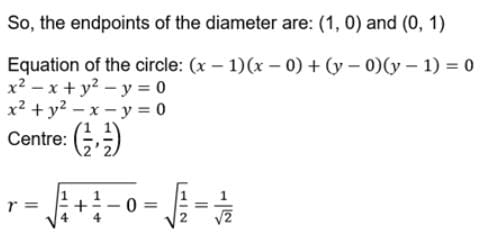Test: Circle- 3 - Question 3

### The length of the tangent from (1, 1) to the circle 2x2 + 2y2 + 5x + 3y + 1 = 0 is

Detailed Solution for Test: Circle- 3 - Question 3

Divide with 2 and apply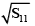Test: Circle- 3 - Question 4

Slopes of tangents through (7, 1) to the circle x2 + y2 = 25 satisfy the equation

Detailed Solution for Test: Circle- 3 - Question 4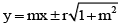and substitute (7, 1)

Test: Circle- 3 - Question 5

The circle with (4, –1) and touching x-axis is

Detailed Solution for Test: Circle- 3 - Question 5

The centre of given circle = (4, -1)

The circle is touching the X-axis.

Then the equation of the circle-

(x-h)2 + (y-k)2 = r2

Where (h, k) = (4, -1)

Since the circle is touching the X-axis. Then

r = k

r = -1

So, (x-4)2 + (y+1)2 = (-1)2
(x2 -8x+16) + (y2 +1+2y) = 1
x2 + y2 - 8x + 2y + 17 = 1
x2 + y2 - 8x + 2y + 16 = 0
This is the required equation of circle.

Test: Circle- 3 - Question 6

The y-intercept of the circle x2 + y2 + 4x + 8y - 5 = 0 is

Detailed Solution for Test: Circle- 3 - Question 6

Point should be on y axis so x=0
y2+8y+5 = 0
y2+8y+16 =5+16
(y+4)2 = 21
y+4 = (21)½
y = -4 +- (21)½
A = (0, 4+(21)½)       B=(0, 4-(21)½)
AB= [(0-0)2 + {(4+(21)½)  - (4-(21)½)}]
= (2(21)½)
= 2(21)½

Test: Circle- 3 - Question 7

The centre of the circle passing through origin and making intercepts 8 and –4 on x and y axes respectively is

Detailed Solution for Test: Circle- 3 - Question 7

Verify g2 - f2 = 12

Test: Circle- 3 - Question 8

Equation of circles touching x-axis at the origin and the line 4x – 3y + 24 = 0 are

Detailed Solution for Test: Circle- 3 - Question 8

Let the equation of circle x2 + y2 + 2gx + 2fy + c = 0..........(1)
Equation of tangent at Origin(0,0)
So, x(0) + y(0) = g(x+0) +f(y+0) + c = 0
or gx + fy + c = 0
But it is given to be axis of x, so y=0
therefore, g = 0, c=0
Equation(1) becomes x2 + y2 + 2fy = 0..(2)
If it touches the line 3y = 4x + 24
i.e 4x - 3y + 24 = 0
Then the length is perpendicular from centre (0,-f) on the line is numerically = radius f,
[4(0) -3(-f) + 24]/(16 + 4)1/2 = +-f
=> 3f + 24 = +- 5f
= f = 12, -3
Putting these values of f in (2), we get
x2 + y2 + 24y = 0, x2 + y2 - 6y = 0

Test: Circle- 3 - Question 9

The equation of the chord of x2 + y2 - 4x + 6y + 3 = 0 whose mid point is (1, -2) is

Detailed Solution for Test: Circle- 3 - Question 9

S1 = S11

Test: Circle- 3 - Question 10

In the given figure, PA and PB are tangents from P to a circle with centre O. If ∠AOB = 130°, then find ∠APB.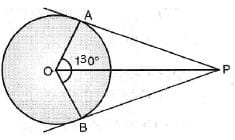Test: Circle- 3 - Question 11

If the two circles (x - 1)2 + (y - 3)2 = r2 and x2 + y2 - 8x + 2y + 8 = 0 intersect in two distinct points, then

Detailed Solution for Test: Circle- 3 - Question 11

C1C2 < r1+ r2

Test: Circle- 3 - Question 12

If the distance between the centres of two circles of radii 3, 4 is 25 then the length of the transverse common tangent is

Detailed Solution for Test: Circle- 3 - Question 12

Length of transverse common tangent = [d2 - (r1+r2)2]1/2
Given d=25 and r1=4 & r2=3
∴ L= [(25)2 - (7)2]1/2
L=24

Test: Circle- 3 - Question 13

The equation of the tangent to the circle x2 + y2 - 4x + 4y - 2 = 0 and (1, 1) is

Detailed Solution for Test: Circle- 3 - Question 13

S1 = 0

Test: Circle- 3 - Question 14

Equation of the tangent to the circle x2 + y2 - 2x + 4y - 4 = 0 which is parallel to the line 3x + 4y -1 = 0 is

Detailed Solution for Test: Circle- 3 - Question 14

r = d

Test: Circle- 3 - Question 15

The equation to the side BC of ΔABC is x + 5 = 0. If (-3, 2) is the orthocentre and (0, 0) is the circumcentre then radius of the circle is

Detailed Solution for Test: Circle- 3 - Question 15

Let D is the image of (–3, 2) w.r.t x + 5 = 0 and r = SD

Test: Circle- 3 - Question 16

The chord of contact of (1, 2) with respect to the circle x2 + y2 - 4x - 6y + 2 = 0 is

Test: Circle- 3 - Question 17

The circles x2 + y2 - 8x + 6y + 21 = 0 , x2 + y2 + 4x - 10y - 115 = 0 ar

Detailed Solution for Test: Circle- 3 - Question 17

C1C2 = |r1 - r2|

Test: Circle- 3 - Question 18

If the circles x2 + y2 + 2gx + 2fy = 0 x2 + y2 + 2g' x + 2f ' y = 0 touch each other then

Detailed Solution for Test: Circle- 3 - Question 18

C1, O, C2 are collinear

Test: Circle- 3 - Question 19

Locus of the point of intersection of tangents to the circle. x2 + y2 + 2x + 4y - 1 = 0 which include an angle of 60o is

Test: Circle- 3 - Question 20

Number of circles touching all the lines x + y – 1 = 0, x – y – 1 = 0 and y + 1 = 0 are

Detailed Solution for Test: Circle- 3 - Question 20

2 liens are parallel

Test: Circle- 3 - Question 21

If the line y = x touches the circle x2 + y2 + 2gx + 2fy + c = 0 at P where OP = 6√2 then c =

Detailed Solution for Test: Circle- 3 - Question 21

Length of tangents from origin

Test: Circle- 3 - Question 22

If the tangent at (3, –4) to the circle x2 + y2 - 4x + 2y - 5 = 0 cuts the circle x2 + y2 + 16x + 2y + 10 = 0 in A and B then the mid point of AB is

Detailed Solution for Test: Circle- 3 - Question 22

S1 = 0 and S1 = S11

Test: Circle- 3 - Question 23

Locus of mid points of chords to the circle x2 + y2 - 8x + 6y + 20 = 0 which are parallel to the line 3x + 4y + 5 = 0 is

Detailed Solution for Test: Circle- 3 - Question 23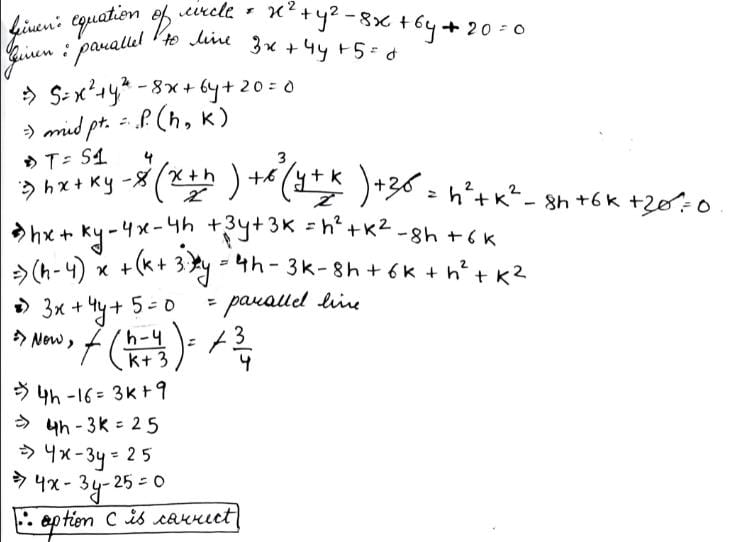Test: Circle- 3 - Question 24

If the circles x2 + y2 = 2 and x2 + y2 - 4x - 4y + λ = 0 have exactly three real common tangents then λ =

Detailed Solution for Test: Circle- 3 - Question 24

C1C2 = r1+ r2

Test: Circle- 3 - Question 25

The locus of midpoints of chords of the circle x2 + y2 - 2x - 2y - 2 = 0 which make an angle of 120o at the centre is

Detailed Solution for Test: Circle- 3 - Question 25

S1 = S11

Test: Circle- 3 - Question 26

If P and Q are the points of intersection of the circles x2 + y2 + 3x + 7y + 2p - 5 = 0 and x2 + y2 + 2x + 2y - p2 = 0 , then there is a circle passing through P, Q and (1, 1) for

Detailed Solution for Test: Circle- 3 - Question 26

Since equation of required circles is S + λS ' = 0
As it passes through (1, 1) the value of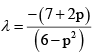If 7 + 2p = 0, it becomes the second circle,
Therefore it is true for all values of p

Test: Circle- 3 - Question 27

Lengths of common tangents of the circles x2 + y2 = 6x, x2 + y2 + 2x = 0 are

Test: Circle- 3 - Question 28

The centre of the circle passing through the points (0, 0), (1, 0) and touching the circle x2 + y2 = 9 is

Test: Circle- 3 - Question 29

The line 2x – y + 1 = 0 is tangent to the circle at the point (2, 5) and the centre of the circles lies on x – 2y = 4. The radius of the circle is

Detailed Solution for Test: Circle- 3 - Question 29

Tangent, T : 2x−y+1=0
Slope of tangent, T to the circle is 2
The line joining the centre and the point of contact of tangent is perpendicular with the tangent.
Thus, slope of line OA = −1/2
Equation of OA, (y−5) = −1/2(x−2)
x + 2y = 12
∴ Intersection with x−2y=4 will give coordinates of centre which are (8,2)
∴r = OA = √(8−2)2 + (2−5)2
=3√5

Test: Circle- 3 - Question 30

Circle with centre (0, 4) and passing through the projection of (2, 4) on x-axis is

Detailed Solution for Test: Circle- 3 - Question 30

Centre (0, 4) and r = 1

## Mathematics (Maths) Class 11

157 videos|210 docs|132 tests
Information about Test: Circle- 3 Page
In this test you can find the Exam questions for Test: Circle- 3 solved & explained in the simplest way possible. Besides giving Questions and answers for Test: Circle- 3, EduRev gives you an ample number of Online tests for practice

## Mathematics (Maths) Class 11

157 videos|210 docs|132 tests(Scan QR code)